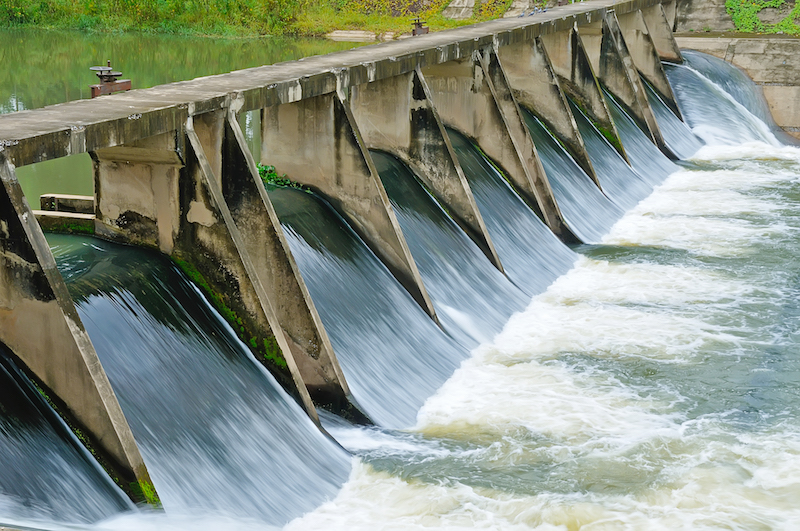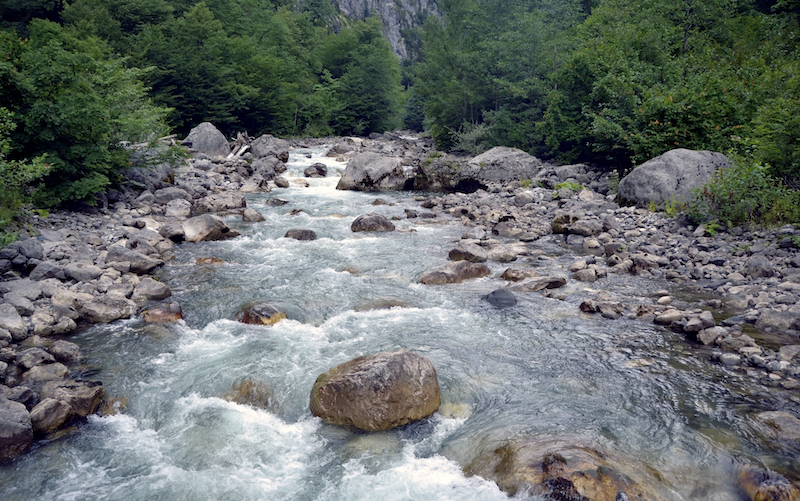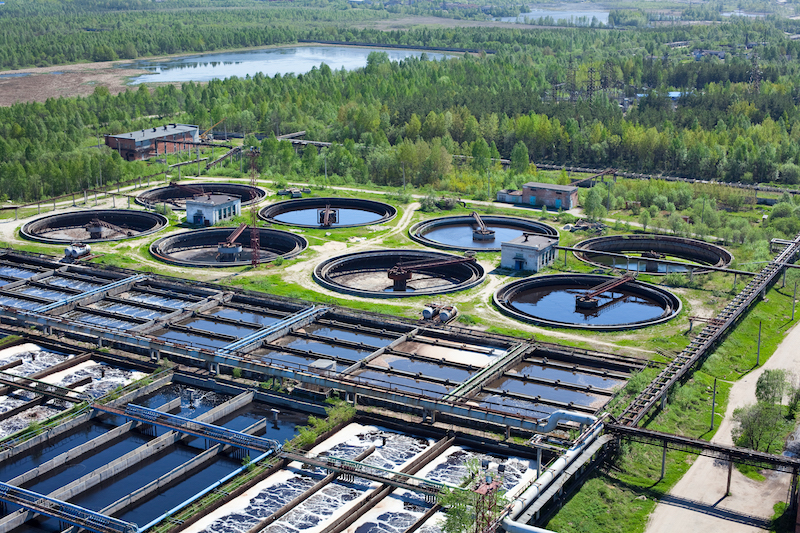Select Page

What is flow? For those of us in the water resources field, it is obvious from our everyday work and experience. But there are some nuances and details about flow that are important to understand.

In this post, I will cover the basic concepts of flow, including some simple ways to understand flow and the various units of flow. I will also cover some more complicated concepts like steady flow, laminar flow, turbulent flow, uniform flow, and critical flow. We will end with an important note about units of flow and a common pitfall when working with some flow units.

#### Basic Concepts of Flow

Flow, or volumetric flow rate, is simply the volume of fluid that passes per unit of time. In water resources, flow is often measured in units of cubic feet per second (cfs), cubic meters per second (cms), gallons per minute (gpm), millions of gallons per day (MGD), or other various units. Understanding flow in water resources is important for applications such as design, operations, system control, billing and many other applications. Flow is often represented with the symbol Q.#### Conversion Factors for Flow Units

Below is a convenient table for use in converting common units of flow. I have also provided some conversion equations for the most common units used. Here is a link to all of the units conversion factors in the H2Ometrics platform.

• 1 MGD = 1.547 cfs
• 1 MGD = 694.44 gpm
• 1 cfs = 0.6463 MGD
• 1 cfs = 448.83 gpmCommon Flow Conversion Units

#### Steady Flow and the Continuity Equation

For water flowing in a pipe under steady-state conditions (i.e., not changing over time), continuity means the water that flows into one end of a pipe must flow out of the other end. This also means that the flow rate in the pipe is the same at any location along the length of the pipe. The continuity equation can be represented as:

Flow = velocity * area

Here is an example of a computation of flow using the continuity equation. The velocity is measured as 10 feet per second and the cross sectional area of the flow is measured as 10 square feet. Flow = 10 feet per second * 10 square feet = 100 cubic feet per second.

#### Laminar / Turbulent Flow

Laminar flow occurs in parallel layers, with no disruptions between those layers. Turbulent flow is chaotic and characterized by flow that contains eddies and swirling currents that occur at random.

Turbulent flow is technically defined by flow that has a Reynolds number (the ratio of inertial forces to viscous forces) that is greater than 1. Almost all flow that we observe in the environment and that occurs in engineered water resource systems is turbulent flow. Even a flowing river that appears very smooth on the surface most likely has swirling currents and eddies beneath.

Because turbulence is chaotic, there are no governing equations that explicitly describe it. This means the equations that we use to represent water flow in most environmental and engineering systems are empirically derived, or based on a statistical curve fit from observations. Because equations and models are empirically derived, they cannot be universally applied. There are times when certain models and equations are appropriate and times when they are not. This is important to keep in mind when performing a complicated hydraulic analysis. It is very important to understand the limitations of the equations being applied and the ranges in which those equations are appropriate.Turbulent flow contains eddies and swirls.

#### Uniform Flow

Uniform flow is a phenomenon that occurs in open channel flow. Uniform flow simply means that the depth of flow is not changing along the length of the channel. This also means that there is a balance between gravity and friction in the channel. Given enough distance away from a hydraulic control structure, the flow will always approach uniform flow depth. The term “normal depth” is sometimes used to refer to the depth in a channel flowing under uniform flow conditions.

The Manning’s equation is an empirical formula that is often used to describe the relationship between the flow and the depth, slope and the channel friction coefficient (Manning’s n) in an open channel under uniform flow conditions. It is common to assume that a channel or conduit is flowing under uniform flow conditions and then to use the Manning equation to derive the flow for a given depth, or estimate the depth that would occur for a given flow.Open channel flow under uniform flow conditions.

#### Critical Flow

Critical flow is also a phenomenon that occurs in open channel flow. Critical velocity in an open channel is equal to the speed that a small gravity wave travels over the water. Critical flow occurs at a Froude number of 1. The Froude number is analogous to the Mach number in airflow, and the notion of supercritical and subcritical flow is analogous to supersonic and subsonic speeds of airflow.Critical flow occurs right at the crest of a spillway like this. Flow upstream of the spillway is subcritical and flow in the spillway is supercritical.

In open channel flow, small gravity waves can travel both upstream and downstream in flow that is traveling slower than critical velocity (subcritical). This means that the hydraulic control in subcritical flow is downstream, like a dam, weir or waterfall. The dynamics of the water passing through such a control structure will control the depth in the channel upstream of the control point. Subcritical flow is common for most slow moving, flat rivers. You can test if an open channel is subcritical by throwing a rock into the water and observing that the resulting waves travel both upstream and downstream.

Small gravity waves can travel only downstream in flow that is traveling faster than critical velocity (supercritical). This means that the hydraulic control in supercritical flow is always upstream, like the crest of a hill, or a discharge beneath a sluice gate. This is common for most fast moving, steep rivers in the mountains. You can test if an open channel is supercritical by throwing a rock into the water and observing that the resulting waves travel only downstream.

In technical terms, critical depth is defined as the depth that results in the minimum specific energy state for a particular discharge. In practical terms, the energy-minimum state means that there is only a single flow rate that corresponds to the critical depth. This is a very useful phenomenon for measuring flows, as it means that only a measurement of depth is needed to determine the flow rate. This is the principle behind measuring flow in a weir or a flume.Supercritical flow in a steep mountain river.

#### A Common Pitfall with Flow Units

Flow is an instantaneous measure of the state of the system. A flow may be at one rate this instant, and at another rate a moment later. This may seem rather obvious given the definition of flow, but sometimes the units used can make this seem counterintuitive.

The time component of the flow units (like MGD or millions of gallons per day) may cause some confusion when these units are used. The pitfall that is easy to fall into is to assume that the measurement must represent an average flow over a day when units of MGD are used. This is not necessarily the case, and units of MGD can still be used to represent an instantaneous measure of flow.

When a measurement of flow has been averaged over a period of time, it is common to indicate that in the description of the flow, not by the units of the flow used. For example, we might say that the average flow for the month of June was 694 gpm. We would not assume that the flow was averaged over one minute just because units of gpm were used. It is understood that gpm is just the units of the flow used, and that the value of the flow rate (694) is the average value for an entire month.

It just so happens that this rate of flow could also be represented as 1 MGD, because 1 MGD is equal to 694 gpm (see conversion rates above). However, just because we represent the flow in units of MGD, this does not mean that the value represents a daily volume or was averaged over a single day. In this example, 1 MGD was the average flow over the entire month of June.This confusion is very common at treatment plants that record daily flow records, and flow units of MGD are used for convenience. For example, imagine that the average daily flow at a plant was 1 MGD on June 1. This means that the total volume of flow that went through the plant that day was 1 million gallons, starting at midnight and ending 24-hours later. However, during the course of the day, the flow certainly varied, and at times was greater than 1 MGD and at other times was less than 1 MGD. The flow rate in MGD can still represent an instantaneous flow value. Units of MGD are often used for daily average flows at a treatment plant as a matter of convenience. But there is nothing unique about the units of MGD that requires the volume to be measured or averaged over an entire day.

#### Flow and H2Ometrics

H2Ometrics provides visualizations, metrics and alerts to continuously monitor flow from any type of flow meter. Using the H2Ometrics tools can help you determine when your flows are doing something that is unusual or different than before, which can help diagnose an issue before it becomes a big problem.

Things like sewer inflow and infiltration, water main breaks, and water quality can all be detected and diagnosed by constantly monitoring your flow data. H2Ometrics does the heavy lifting for you by providing tools that make it fast and easy to generate actionable output from your data.